AsTher Thermodynamic Database and Applications

AsTher Process Calculator for MS Excel

The application is very simple to use but with powerful performance
for calculation of the equilibrium state, as well as for create of process modelsContent
1.General
2. Definition of the Thermodynamic System and Calculation
2.1. Definition of the phases of the thermodynamic systems
2.2. Selection of the Substances in the Phases from the AsTher Database
2.3.Definition of the Calculation Conditions
2.4. Execute Calculation
3. Possible Errors and Trouble Shooting - Behaviour of Process Calculator  and MS Excel
4.1. State functions Cp, H, G, S and Energy Balance
4.2. Element Balance
4.3. Inverse calculation of an equilibrium state
4.4. Data capture and recording using calculations
4.5. Graphic chart of the calculation results
4.6. Visual display of the calculations results
4.7 Process Calculator as Macro: Parameter Definition and Create Parameters for Macro Calculations
5. Examples
5.1. Quick Start: Create MS Excel File (Book) for calculations using AsTher Process Calculator for MS Excel, Step by Step
5.2. CH4-Combustion, Simple calculation using AsTher Process Calculator for MS Excel, an example for simple calculations
5.3. Carbon gasification by Water in depends of temperature and pressure, Create graphics using different parameter.
5.4. Enthalpy-Concentration, Liquid-Gas-Concentration-Diagrams of Ethanol-Water Mixtures using empirical activity coefficients.
5.5. Example for inverse Calculation using AsTher Process Calculator for MS Excel
5.6. Aqueous substances, solubility and heat of solution

1. General

Process Calculator for MS Excel (XProCalc.exe) calculates thermodynamic equilibriums corresponding to the input data in a MS Excel Sheet (Table), writes the calculation result in the same MS Excel Sheets and supports MS Excel Versions 97 until MS Excel Version 20XX for 64 bit operations systems.

Important functions of the user interface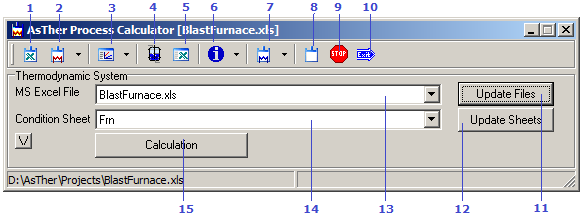1..Create Application creates a new MS Excel Book as thermodynamic system 2. Submenus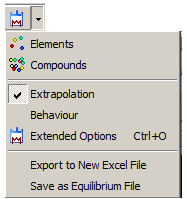Elements: selection of the elements for thermodynamic system Compounds: system selection of the substances for thermodynamic system, before "Condtions Sheet" is the phase sheet of the system. Extrapolation : Enable extrapolation of the thermodynamic date (extrapolation is often recommandet) Behaviour : Options of the behaviour of the thermodynamic system Extended Options: calculation options Export to New Excel File: the thermodynamic system is exported in an new MS Excel book without linked data. (new files will be independent of the source file) Save as Equilibrium File:  the thermodynamic system is exported in an AsTher Equilibrium-File 3. SubmenusState Function: Values of the state functions are written in the phase sheets of the thermodynamic system Element Balance: Elementary balance of the thermodynamic calculations is written in a new MS Excel Sheet depends of calculations. When a calculation is new carried out, then elementary balance is updated. Lagrange Coefficients of the elements in the thermodynamic system 4.Visualisation Visual display of the calculation results in a picture 5. Show MS Excel MS Excel Book is shown, when the current system is hidden in display 6.SubmenusMessages: shows application messages Help: show help files Registration: Software registration informations About: Software registration informations 7. Submenus Execute external application of AsTher Pure Substance Reaction Database Administration Shows the project directory in MS Windows Expolorer 8. Reset Deletes calculationresults in MS Excel File 9. Stop the current calculation is broken 10.Close Close application 11. Update list in (13) Update the list  of the MS Excel Books in the field (13) , which are open 12..Update list in (14) Update the list of the sheets  in the field (14) of the selected MS Excel Book 13. MS Excel Books List of the MS Excel Books, which are open 14. Sheets List of the sheets if the MS Excel Books in the field (13) 15. Calculation Calculation of thermodynamic equilibrium, which are defined in the sheet in (14) of the file in (13)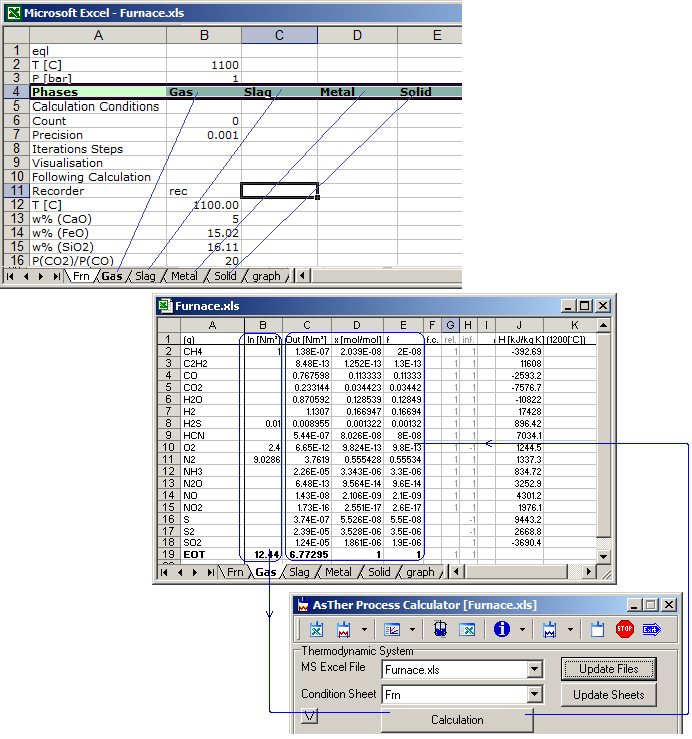In the example above, the thermodynamic system is defined in the sheet Frn.
The thermodynamic system contains 4 phases: Gas, Slag, Metal, Solid, which exists as separate sheet.
Input data are given in column [B] in the phase sheets.
Calculation results is written by application in the columns [C], [D],[E] . .  in the phase sheets.
The Excel sheets are input and output file of the calculations.
MS Excel must be installed in your computer.

When the application is executed without parameters, then you have already to open an MS Excel file,  and you can select an open file, as the example above shows.

Several Applications for process simulation and process control use MS Excel tables for data input/output.
Most of the Applications for the simulation can not calculate any equilibrium state.
Using MS Excel interface, you can integrate Process Calculator for MS Excel (XProCalc.exe) into the software for process simulations, and calculate equilibrium states.

You can calculate also as macro, but you can calculate without macro or as single application.
When you deactivate macros in MS Excel, you can also run Process Calculator for MS Excel using a symbolic link or in another external program.

2. Definition of the Thermodynamic System and Calculation

2.1. Definition of the phases of the thermodynamic systems

Every phase must be defined in a separate sheet.
The following picture shows, how a phase is defined in the thermodynamic system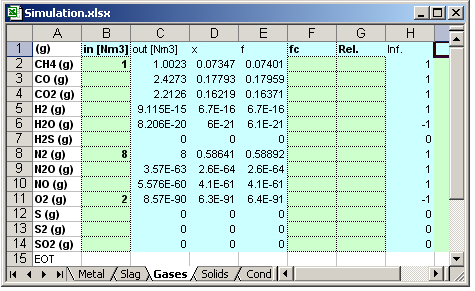Column A:
Cell A1, the state of the phase is given as: (g), (l), or (s)
The cells A2 to A256 contains substance names
After the last substance, the tables should end EOT. All data behind the cell EOT are not regarded.

Column B: contains input values. In the cell B1, the dimension should be given.
Valid values are: in [mol], in [kg], in [g], in [lb] and for gases also in [m³], in [Nm³], in [ft³]
When no any valid dimension is given, the dimensions are regarded as [mol].

Column C: contains output values as calculations result.
In the cell C1, the dimension should be given.
Valid values are: out [mol], out [kg], out [g], out [lb] and for gases also out [m³], out [Nm³], out [ft³]
When no any valid dimension is given, the dimensions are regarded as [mol].
All Cells C1 to C(until EOT) are overwritten, when a calculation succeed.

Column D: contains output concentration values as calculation result.
In the cell D1, the dimension should be given
valid values: x, w, w%;
x
means mol ration,
w is weight ratio,
w%
is wait ratio as per cent.

When no any dimension defined, the default output concentrations are in mol ratio.
All Cells D1 to D(until EOT) are overwritten, when a calculation succeed.

Column E: contains fugacity (f) of gas substances or activity (a) of the solid or liquid substances.
All Cells E1 to E(until EOT) are overwritten, when a calculation succeed.

Column F: Activity or fugacity coefficient of a substance.
When the coefficients are known, you can enter values.
When no any value is given, the value is regarded as 1.
The Cells G1 to G(until EOT) is not overwritten by application.

When a substance can be exist only as pure substance, like soot in combustion processes,
enter  -1 or  '(1), (number one in parenthesis or Some times may be apostrophe necessary)

Column G: Mass relation of the input values, which reacts in the equilibrium system.
valid values: 0 to 1.
When no any value is given, the value is regarded as 1.
When an invalid value is given, the value is also regarded as 1.

When in column B is written 10 kg for a substance for initial mass and in column G is written 0.25
then the reacting mass is 2.5 kg. the residual mass of 7.5 kg is exists in products without reaction.

Column H. Information of the formation of substances
1: system  is conveniently for formation;
-1: Formation is inhibited ;
0: formation is is disabled or inpossible.
(ext): thermodynamic data are extrapolated.

2.2. Selection of the Substances in the Phases from the AsTher Database

You can enter substances in a phase sheet manual. Phase format is given in cap. (2.1.).
You can also select substances from database as following

When you execute XProCalc.exe without parameters or using parameters, press button for thermodynamic system, as the following picture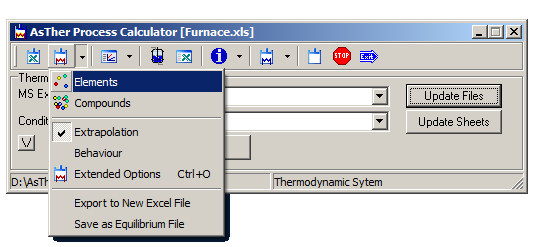In the following dialog box, select the elements of the requested substances pressing the buttons for the elements. The selected elements are shown in red colour.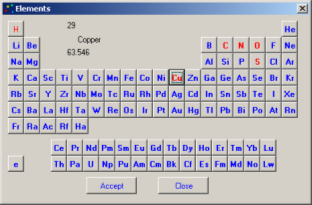Select the elements of the thermodynamic Press button Accept .

2.2.1. Compounds Selection:
Select a sheet for Conditopn Sheet, in which is defined the thermodynamic system, in following picture Frn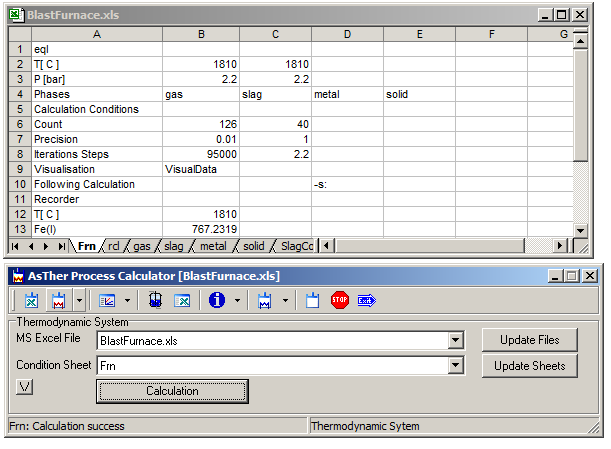In the main window, press drop down menu [Compounds of ->] and in the Submenu select a Phase ( in the following picture like [slag] ).
The application creates the submenus for phase composition (for gas, slag, metal, solid, . . .) corresponding to the entries for phases in the Condition Sheet  ( in the picture above [Frn] ). .The menu "[Compounds of ->]" is visible, when the selected "Condition Sheet" is a thermodynamic system definition

Left list shows substances in the database. Left list shows already selected substances.
You can add substances by mouse double click or using button [ Select -> ].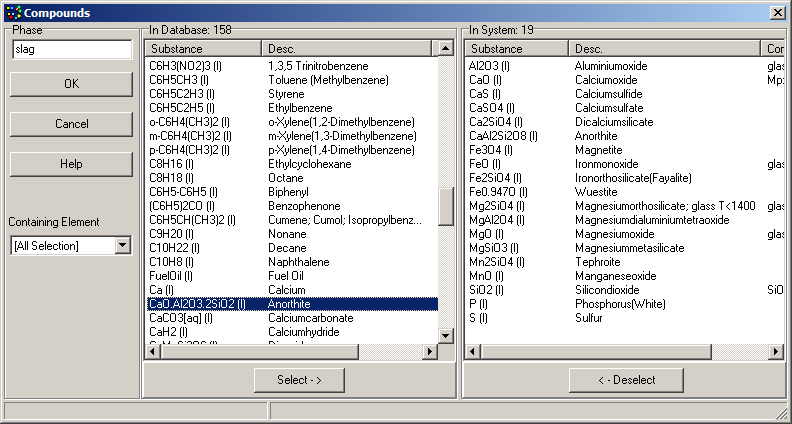When the selection is complete, then press Button [ OK ]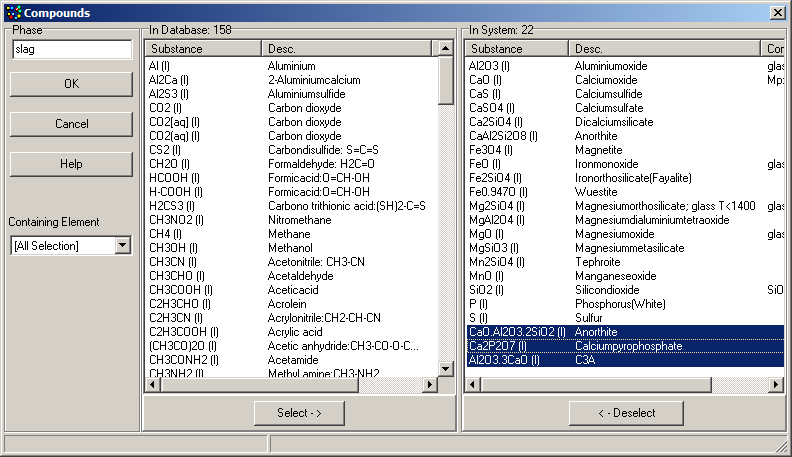The new selections are added in the phase sheet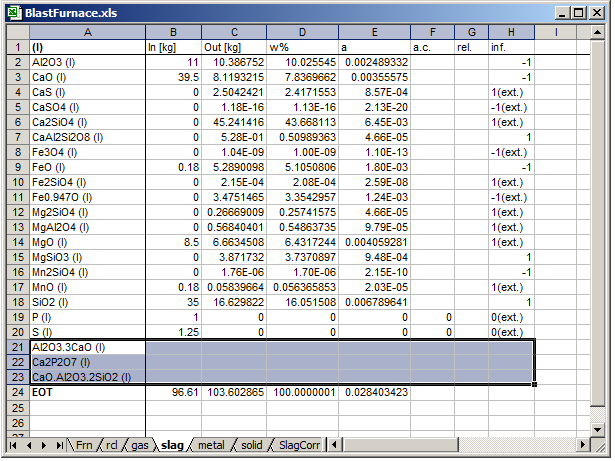The Application never removes a substance from MS Excel tables.
You must remove substances self in the MS Excel sheets, if you want to remove a substance from thermodynamic system.

2.2.2. Clone Compounds Selections:
When you substance selected in a liquid phase like FeO (l), CaSiO3 (l), MnO (l) . . .,
and when you want to select the same substances in a solid phase as FeO (s), CaSiO3 (s), MnO (s),
then select the source phase in the main window as following pictureYou can insert the same substances in another phase using Menu item From [source phase] to a [destination phase].
All substances are added in the another phase, when the substances do not already exits.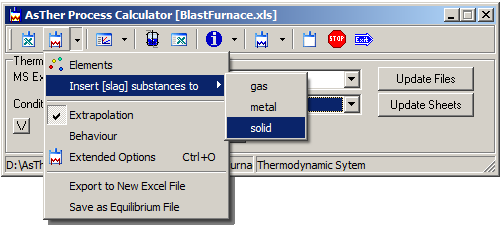The menu " Insert Substances" is visible, when the selected "Condition Sheet" is a phase definition.

2.3. Definition of the Calculation Conditions

The calculation conditions temperature and pressure have to be given in a sheet.

A1; eql: calculation conditions for thermodynamic equilibrium
In the Sheet for the condition, in the example in the below picture  FurnCond,
B2 ,Temperature value, when dimension of the temperature fails  in B1, then °C is valid for temperatures.
B3, Pressure value is in [bar] for const Pressure or Volume [m³] of a gasphase.
When volume is given, then the sheet name of the a gas phase must be given cell B4 (the first phase must be a gas phase).
B5, Calculation Conditions:
Accepted data: s or g; other values are ignored
g: the solid and liquid phases exist in a system, and the phases separated clearly.
Example: a liquid metal and a liquid slag phase exist in a not-turbulent system. For each phase is: Σai ≈ 1
s: recommended, when the calculation is process model of a turbulent system.

Example: Solid phase and/or liquid metallic phase dispersed in a liquid slag phase.
Calculations using parameter s are based on experience in process modelling of metallurgical processes.
The calculation is carried out for the possible highest entropy of the system, Not in every phase Σai ≈ 1
B4..I4 Phases in System :
the sheet names are given by users in the cells, which are regarded in the calculation.
The sheets must exist in the same MS Excel file.
The system can contain until 8 Phases, there are until 2 gas-, until 3 liquid- and until 3 solid phases can be given.
When the calculation is succeed, then the text "Success" is written by XProCalc.exe in the cell B1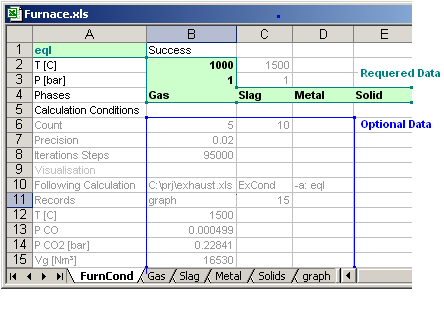The data below the Row 5 are optional parameters

B6, Count: When a calculation executed, the count of the calculation is written in the cell B5;
When the number in the cell C6 is higher than the calculation count, then a following calculation is executed.
The MS Excel file for the following process can be defined in the cell B10.
The Following Process may be defined in the same MS Excel File or in an different MS Excel File.
When you use the same MS Excel file for more than one processes, the conditions should be defined in the separate sheets for each process..

B7 Precision of the calculation result.
The precision should be less than 0.1.
A precision of 0.05 is sufficient.
Default value is 0.01.
Range is: 0<[B7]Value<1.

The multiplication by 100 of the given precision value yields the precision value in per cent,
The value 0.02 means a precision of 2%.

When you make a process simulation, the precision value should be not less than 0.02.
When you calculate an equilibrium state, the value should be less than 0.02.

An exact calculation of the equilibrium state often causes  higher difference of the real systems.
When a process simulation is intended. The value of the precision should correspond nearly to the precision of the inputs values.

B8 Number iteration steps: The thermodynamic equilibrium is calculated iterative.
When no any solution is found until the given iteration steps, the calculation is broken.
The values should be less than 100000.

B10: The Filename and the sheet name for the conditions of the following calculation is given in Cells B10 and C10.
File Name can be given full path
|C:\MyDocuments\Projects\Calculation\system2.xlsx|

or relative path to the current file like following.
|..\OtherProjects\system2.xls|

when the file is already open, you can enter only the filename without path
|System2.xls|

When you enter "*", the following calculation is in the same MS Excel file

C10: Condition sheet of the following calculation
When you enter "*", the condition sheet of the  following calculation is in the same Sheet.

Such calculation is useful, when one ore more conditions or input data  in the thermodynamic system is changed in depend of the calculations count.
Following calculation is run, when the value is more than zero in the cell C6

The following example shows, that the temperature is varied from 300°C to 1000°C by steps of 100°C in depend of the calculations count in the cells B6 and C6. 8 Calculations are carried out.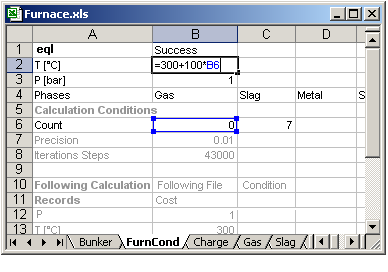The following example shows, that the systems in the files Furnace.xls and Cleaning.xls are calculated.
A Following calculations can be carried out, when the value in B6 is equal to C6 or less than C6 .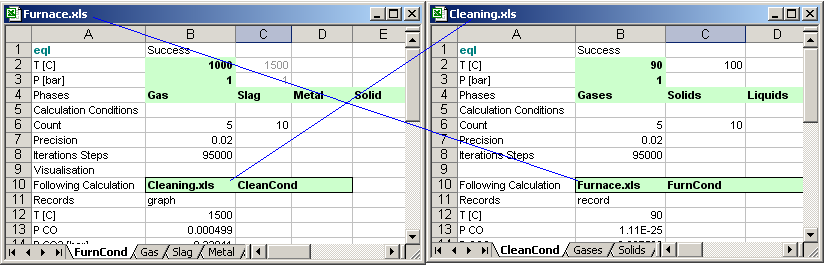B13, Sheet name for data records defined in the Cell B13
When you calculate varying parameters, you can record the selected data in the given sheet name. in the cell B13.
After each calculation, the data in the cell B14 and the bellows B14 is written in the sheet for data record in the rows corresponding to the calculation count.

.In the example above left , the sheet for data record is in the sheet "graph"
In the example above right, the sheet for data record is  in the sheet "record"
4.3. Data capture and recording using calculations
4.4. Graphic chart of the calculation result

Important for macro, calculations using link or using MS Windows API command ShellExecute, ShellExecuteEx:
When XProCalc.exe executed using parameter, then the condition sheet name is given after the parameter -c:
Example:
-x: C:\AsTher\projects\Furnace.xls -c: FurnCond

2.4. Execute Calculation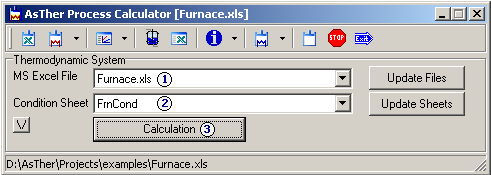When Process Calculator for MS Excel runs, in the main window
(1): Select MS Excel file
(2): Select the Condition sheet
(3): Press button Calculations

Calculation results are written in the columns for calculation thermodynamic equilibrium:
C: output values
D: output concentrations
E: Activity or fugacity

3. Possible Errors and Trouble Shooting

3.1. Check List, when a calculation is not carried out

Thermodynamic system must be defined in a sheet in MS Excel, see  also 2.3. Definition of the Calculation Conditions3.1.1. In the cell [A1], eql or rcl  must be written.
eql: calculation of an equilibrium
rcl: inverse calculation of an equilibrium state

3.1.2. Temperature and pressure have to given in the cells [B2] and [B3]

3.1.3. Thermodynamic system must be contains at last one phase.
The phases are defined in separate sheets.
The sheet names of the phases are written in the cells [B4], [C4], [D4] . . . [I4].
Maximum 8 Phases are permitted.
When a compound exists as pure substance, a separate sheet is not necessary,  you can enter in the column for ac [F]  -1(minus one)
In the Cell [A1] in the each sheet, the state must be given, (g) or (l) or (s).
Phase sheet must be end EOT
3.1.4.
All errors are written in the message window

3.2. Behaviour of the MS Excel

When MS Excel was not open by user and Process Calculator is executed using parameters,
then Process Calculator opens MS Excel in background.
The calculation results are written in the MS Excel file, the file is saved und MS Excel will be closed.

When MS Excel was already open by user, then Process Calculator shows the given MS Excel file in the active MS Excel Window.
The results are written in the MS Excel file but the file is not saved by Process Calculator.
When you are going to close MS Excel, you will be asked by MS Excel, if you want to save the changes.

3.2.1. MS Excel does usually not permit more than one access at the same time.
When you edit a cell  in a sheet (when a cell gets focus), the application Process Calculator for MS Excel (XProCalc.exe) can not read or write in the MS Excel file. When you are editing a cell the MS Excel file and execute XProCalc.exe, then an error can be occurred.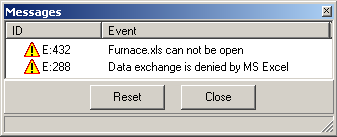When automatic save in every (i.e. 10 minutes) is activated in MS Excel (MS Excel Menu Extras -> Options) , read and write is not possible while saving.
You can see error massage, that the data exchange not possible.

3.2.2. When more than one MS Excel applications run.
Several MS Excel Versions ore Multiple Document Application. You can in a MS Excel Window more than one MS Excel File open. Following picture shows two MS Excel windows. XProCalc.exe can access to only one instance of the MS Excel windows. You can see in the Task Manager of the MS Windows, how many MS Excel windows are open.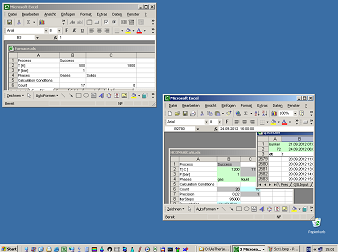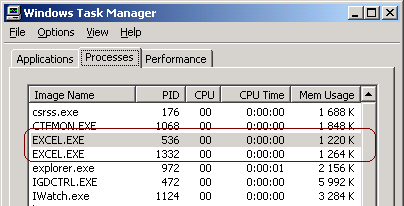Execute Process Calculator for MS Excel (XProCalc.exe) and press button to show the MS Excel, you can see, which MS Excel Window (instance) is accessed by Process Calculator for MS Excel. The MS Excel window can be shown, even if the window was hidden.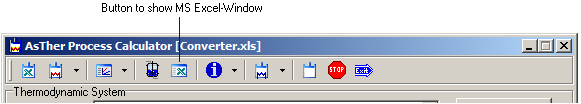3.2.3. When no any MS Excel window is open
When you execute XProCalc.exe using parameter as following,
-x:C:\AsTher\projects\Furnace.xls -c: FurnCond -s:
and no any MS Excel window is open, XProCalc.exe execs MS Excel in the background, you can not see
Furnace.xls

You can avoid the problem, when you execute MS Excel before  XProCalc.exe is executed.

3.3. when the mass-conservation is not possible, the calculation will be terminated. For example, if elementary oxygen (O2 (g) or O (g) ) does not exist ina system consisting of the elements H and O, when only the substances H2(g) and H2O(l) for the calculation selected, the calculation is impossible for 1 bar and 1000 °C. Calculations are broken .
To avoid this, it is recommended to include first time all possible substances into the calculation. You can deselect substances from the calculation system, when it is secure, that the deselected substances can not be exist in the system.
Height number of substances causes long calculation time and imprecision. The thermodynamic data set of  the rare substances may be some times approximated. It is also difficult to overview.
Low number of substances causes fast calculation time. It is easy to overview. But the calculation may be incorrect, because the system  may be not complete.
An optimum is often individual to decide.

3.4. Error messages related to operations system may occur due to excessively high values of the free energy of a substance, so that the processor can not interpret the value. In this case, please check the thermodynamic data set.

3.5. Two reaction systems with high different quantities may produce erroneous results.
Example, when a system consisting of Fe, Cl2, FeCl2, H2O,CO,CO2,N2,NO.
wherein one reaction system is consisting of Fe-Cl2-FeCl2 and  the other consisting of C-CO-H2O-N2-NO.
If the inputs of the calculation is 10000 mol FeCl2, 1 mol H2O and 1 mol N2, 0.1 mol C, and the precision is 0.01,
the calculation result may be incorrect for the system C-CO-H2O-N2-NO.
It is recommended to calculate in separate systems.

3.6. Pure Substances :
If a substance my be exists as a pure substance (and accordingly the activity coefficient has been set to (1) in the a.c./f.c.- column, please check after the calculation, whether:
- the info column shows the value 1,
- the amount of that substance is more than 1e-23 mol,
- the calculated value or the activity is nearly 1.
If not, those substances should be excluded from the calculation, e.g. by setting the a.c.-value to 0.

3.7. An Obviously Erroneous Result (e.g. the formation of liquid Fe at 20 °C), although the calculation results keeps consistent.
This may happen, if the extrapolation is enabled and the extrapolated data itself are unusable.
With the application Pure Substance, you can follow the course of the free Enthalpy in graphic and table window in order to check the validity range of the required dataset.

3.8. Erroneous calculation can be caused by the incomplete definition of the system. The system can be influenced by mass of the gaseous substances, although these substance can not take in any reaction. The results of the calculation will be is different in a system consisting of CaO, CaCO3, CO and N2, when the mass of N2 is different, although N2 does not react.

4.1. State functions Cp, H, G, S and Energy Balance

You can get the values of the state functions in the column [I] and/or [J].
When you enter in the Cell [I 1], [J 1] one of the following chars,
For Specific heat: Cp or  Cp[J/mol K] or  Cp[J/g K] or  Cp[kJ/kg K] . . .
For Enthalpy:       H or  H[J/mol] or  H[J/g] or  H[kJ/kg] . . .
For Entropy:         S or  S[J/mol K] or  S[J/g K] or  S[kJ/kg K] . . .
For  Free energy: G or  G[J/mol] or  G[J/g] or  G[kJ/kg] . . .
For Mol weight:   M or  M[g/mol]

The temperature and pressure of the state functions corresponds to the value in B2, B3 in the conditions sheet,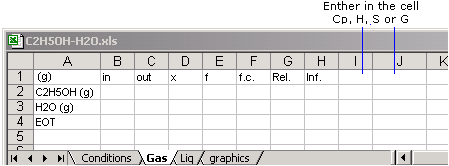The values of the state functions are written in the columns I and J after each calculation.
It is useful, when the temperature of the thermodynamic systems is changed during calculations.

The values of the state function is written only one time in the column [K] and after [K], as the following picture shows
(1) Main window Menu, select State functions
(2) In the following dialog box Select state function, dimension and column to write
(3) Press button "Set"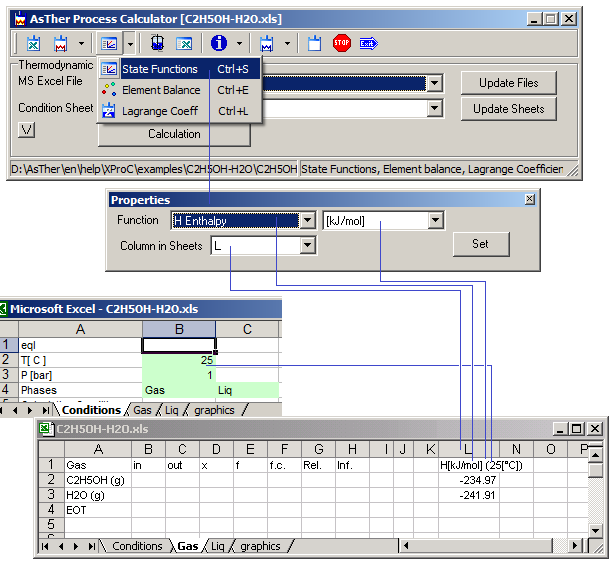when no any value exists in the cells I2 to I256.
You enter another value for the temperature in the cells I2 to I256.
When you cut the values from J and paste into columns K or after K, the calculation time will be faster, because the data write/read in MS Excel files costly in terms of time

Corresponding to the data of enthalpy and the mol weight, you can calculate heat balances.

4.2. Element Balance
the distribution of the elements in the substances of the thermodynamic system can be shown in a new MS Excel book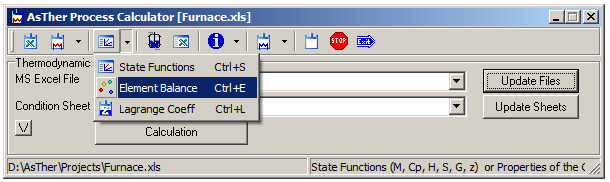4.2.1. Press button for Element Balance, a dialog box will be shown, in which the element can be selected to balance.
When the button Create Balance is presses, a new MS Excel Workbook is created by application Process Calculator for element balance.
The new created book contains links to the MS Excel book, in which is the calculation of the equilibrium state is carried out.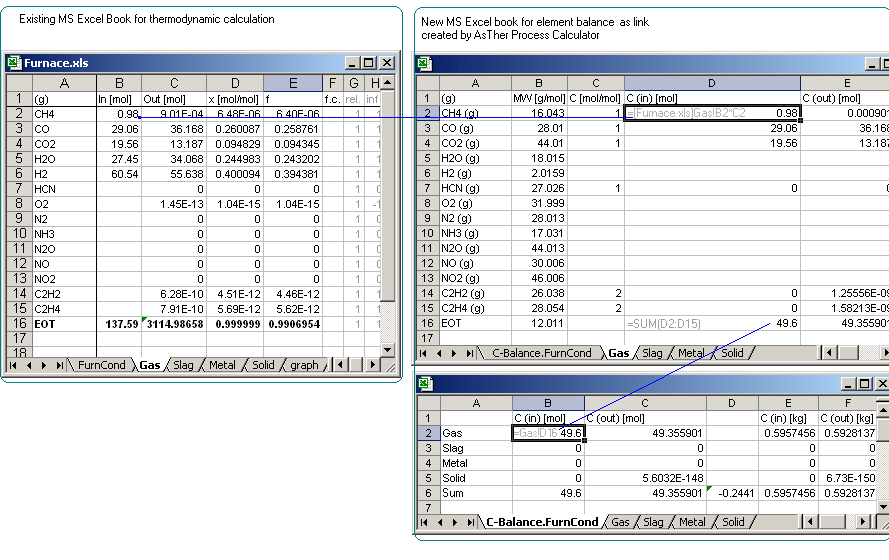4.2.2. Possible errors in element balance
The dimension for In-Columns  and Out-Columns should be given as [g], [kg], [lb] or [Nm³] in the thermodynamic system.

When the dimensions for In-Columns or Out-Columns are given [l] (liters), [m³] or [ft³] and,
when the temperature of the thermodynamic system is changed, then the element balance is not correct.

The calculated values for input and outputs in the element balance depends of the temperature and pressure of the thermodynamic system,
when the dimensions are [l] (liters), [m³] or [ft³] .

4.3. Inverse calculation of an equilibrium system

When several values of the concentrations are known, the application calculates the other concentrations in an equilibrium system corresponding known concentrations. The possible temperature and/or pressure can be estimated in an equilibrium state corresponding to given conditions or  measurements.

When the concentration of the CO2, H2O, N2, NH3 are known as reference measurement in a thermodynamic system consisting of the elements C, O, H, N, the concentration of the other substances with the system elements (like CO, N2O, NO, HCN, CH4 etc.)  can be determined.See also Example for inverse Calculation using AsTher Process Calculator for MS Excel

4.3.1. Conditions Sheet
[A1]
must be written "rcl". rcl means, that the conditions are used for inverse calculations (recalculation of the thermodynamic system)
The temperature und pressure are written in the condition sheet in the cells [B2] [B3]
Sheet names for the phases of thermodynamic system are written in the cells [B4], [C4], [D4]... [I4],
When no any entry exits in the calls [B5], [C5], [D5], ... [I5],
then:
Column [E]: Measurements or the known concentration, activity, fugacity of the substances are read by the application .
Column [K]: "x" is written, when the corresponding value in column [E] used as reference.
Column [L]: Calculations results are written by application.

You can use another columns for the calculation, when you write the column letter in the Cells [B5] to [I5],
bellow the sheet names of the phase
The letters BDE means:
Colum B in the phase sheets contains values of the references or measurements
Colum D in the phase sheets contains, which references or measurements are to use for the calculation
Colum E is to write the calculation results
When no any data entered, default value is EKL
When DKLM is written, then in the column M is written, if the thermodynamic data is extrapolated.

7.3.2. Phase Sheets
When a concentration or fugacity value in column [E2] to [E256] conducts to reference,
the letter "x" must be written in the corresponding cells [K2] to [K256] in the phase sheets
Calculation results are written in the column [L2] to [L256]

4.3.3 Calculation
when button Calculation is pressed, then the calculation will be carried out
When no any data is written in the cell [B5], [C5],[D5]... [I5],
Default values are:
Column E: are measurement;
Column K: contains references indicators "x";
Column L: calculation results
Column M: information

In the example bellow, the measurements are given in column D.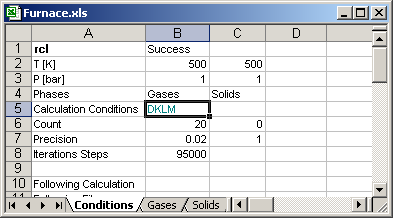Calculation Results are written as following.
Green cells: given data by user for the calculation,
Blue cells: written by Process Calculator as calculation result.
The concentration value of  Cl2 (g) [D6] used as reference measurement, because the cell [K6] contains "x",
calculated value is written in the cell [L6]. In the Cells [M6] is nothing written by application,
because thermodynamic data of Cl2 (g) is not extrapolated.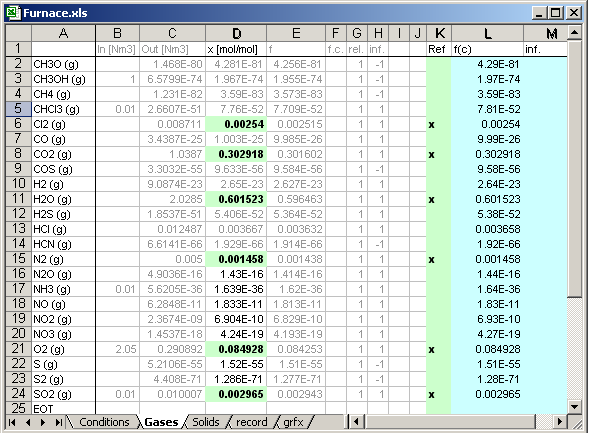4.4. Data capture and recording using calculations

When you calculate the system and vary parameters, you can record the defined data.
The record variables must be given as link, as the following example shows.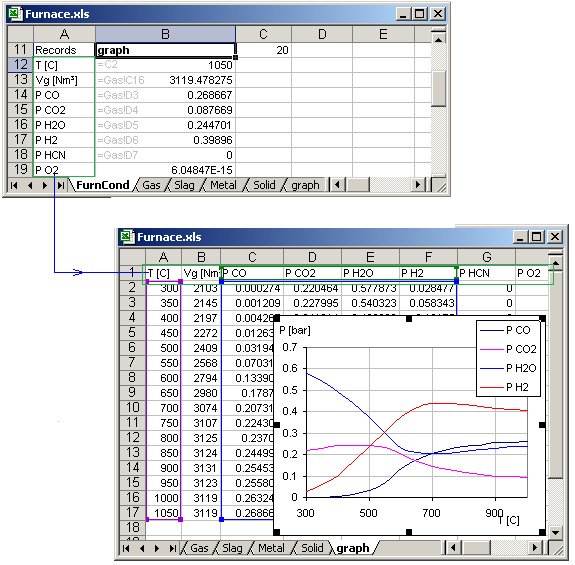In the example above:
Variable names are given by user in the cells from [A13]  to [A20].
Variable values are written by user as link in the cells from [B13]  to [B20].

The definition of the graphic is leaved to user. The rows of the written data in the Record-Sheet (in the example above  graph) always correspond to the calculation count in the cell B6 of Conditions-Sheet.

When the calculation count is 0 (zero), then the cells A13 to A20 are written by application in the row A1, A2, .. A8 in the sheet "graph" (Because graph is given by user in the cell cell [B12])

4.5. Graphic chart of the calculation result

Creation of the graphic is leaved to user. The application AsTher Process Calculator for MS Excel does not make graphics.
Following link shows an example for graphics

4.6. Visual display of the calculations results

You can display significant data of the calculations in a window.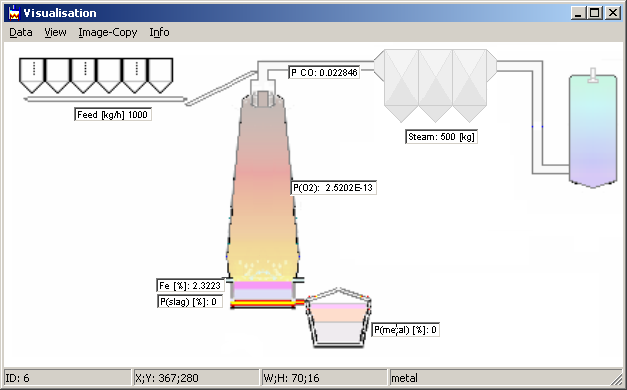Data are to write given format in a sheet. The sheet name is given in the [B9] of the conditions sheet.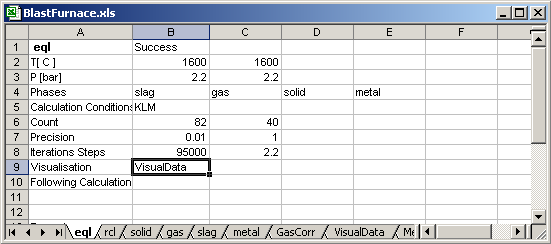Column A In the Visualisation sheet
I: Picture name for back ground. Default no any picture
F: Font name. Font size is given in column B, Default system Font
W: Position of the Visualisation-Window on screen given in column E,F,G,H for top, left, width, height. Default no any fixed position.
1....96: Field ID the in the Visualisation-Window.

Column B,C

The Fileds in the visual window contain the strings from [Bn] and [Cn], where n: 1..96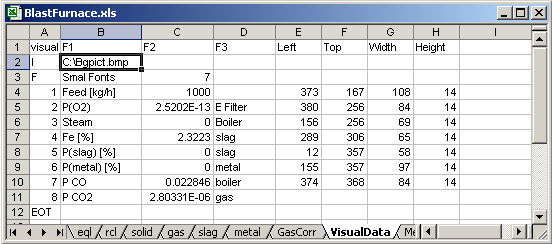Column E, F, G, H
Left:
distance of the Field from left site of the window in pixel.
Top: distance of the Field from top site of the window in pixel.
Width, Height : Field dimensions in pixel.

4.7.Calculation Using Parameters or Macro

When the application XProCalc.exe is executed using parameters without -s:, the application closes immediately after the transfer of the calculation result to MS Excel file. You can see only the icon in the MS Windows Task Bar and the calculation progress, while the calculation runs.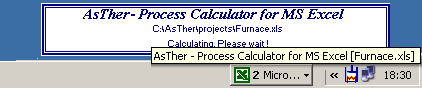The application XProCalc.exe can be executed also with defined parameters, like following example shows.
The parametric calculation carried out with the MS Windows commands ShellExecute or MS Excel Macro Command Shell

Shell("C:\Programs\AsTher\bin\XProCalc.exe -x: C:\AsTher\Projects\Furnace.xls -c: FurnCond -s:")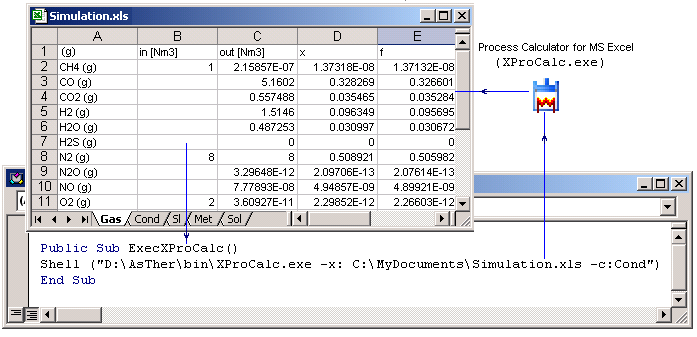Valid parameters are

-x: is always the first parameter. The name of the MS Excel file follows  -x:
Blanc character or specific character should not be used in the file name.
The application breaks calculation, and shows error message, when the file does not exist.
Parameter -x: is necessary.

-c:  is always the second parameter. After -x:, the name of the sheet is given, which contains the calculation conditions,
temperature, pressure, and the name of the sheets, which contains the thermodynamic system.

-r: Read only, The calculation will be executed, but no any cell in the MS Excel file is written after the calculation.
The application is not closed.
When  the parameter -r: exits, then the parameter -s: takes no any effect.
Parameter -r: is optional

-s: Process Calculator for MS Excel is usually closed, when a calculation is executed using parameters and succeed.
The application will not be closed, when the parameters contain -s:, even if  a calculation is succeed.
You can see messages of the application.
Parameter -s: is optional

-t: Process Calculator for MS Excel is usually not closed, when an error occurs, independent of the parameter -s: is given.
You can force the application to close after a calculations using parameters, independent of the calculation succeed or an error occurred.
When you enter parameter "-t: 15", the application shows calculation state for 15 seconds before termination.
Parameter -t: is optional.
When  the parameter -t: is given, and if an error occurred, you my not directly see the error messages.
When the MS Excel book can not be open.
You have to check the calculation count before and after the calculation in the Condition-Sheet [B6].
When the calculation count is the same before and after the execution using parameters, then no any calculation is carried out.

When the Process Calculator for MS Excel (XProCalc.exe) executed without parameters, a widow is shown.
In the window, you can create the parameter string with mouse clicks.

When the Button "Search" is pressed, in the following dialog box, you can select a MS Excel file.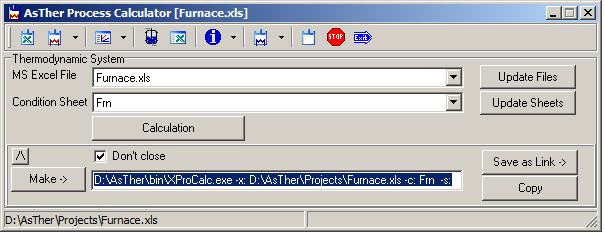After the file selection, the sheets names of the selected Excel file are shown in the selection box.
In the selection box "Conditions Sheet", the sheet is to select, which contains the calculation conditions.

Button "Make ->" creates the parameter string.

Button "Copy" the parameter string is copied to the clipboard.

Button "Calculation" runs immediately XProCalc.exe .
When Read only is not selected, the calculation results are written in the MS Excel File.
Important: When you do not select "Don't Close" the application is terminated immediately after the calculation, when the button "Calculation" is pressed and when no errors are occurred.

Button Help, the help file will be shown.

Button Exit: Only the window is closed. The application runs, and you can see the application icon in the taskbar.